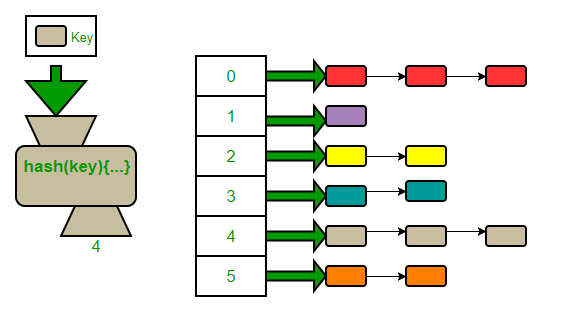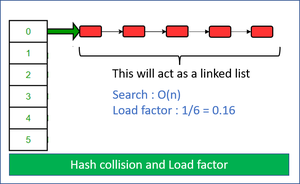Open In App

# Implementing our Own Hash Table with Separate Chaining in Java

All data structure has their own special characteristics, for example, a BST is used when quick searching of an element (in log(n)) is required. A heap or a priority queue is used when the minimum or maximum element needs to be fetched in constant time. Similarly, a hash table is used to fetch, add and remove an element in constant time. Anyone must be clear with the working of a hash table before moving on to the implementation aspect. So here is a brief background on the working of a hash table, and also it should be noted that we will be using Hash Map and Hash Table terminology interchangeably though in Java HashTables are thread-safe while HashMaps are not.

The code we are going to implement is available at Link 1 and Link2

But it is strongly recommended that one must read this blog completely and try and decipher the nitty-gritty of what goes into implementing a hash map and then try to write the code yourself.

Background: Every hash-table stores its data in the form of a (key, value) combination. Interestingly every key is unique in a Hash Table but values can repeat which means values can be the same for different keys present in it. Now as we observe in an array to fetch a value we provide the position/index corresponding to the value in that array. In a Hash Table, instead of an index, we use a key to fetch the value corresponding to that key. Now the entire process is described below

Every time a key is generated. The key is passed to a hash function. Every hash function has two parts a Hash code and a Compressor

Hash code is an Integer number (random or non-random). In Java, every object has its own hash code. We will use the hash code generated by JVM in our hash function and compress the hash code we modulo(%) the hash code by the size of the hash table. So modulo operator is a compressor in our implementation.

The entire process ensures that for any key, we get an integer position within the size of the Hash Table to insert the corresponding value.

So the process is simple, the user gives a (key, value) pair set as input, and based on the value generated by the hash function an index is generated to where the value corresponding to the particular key is stored. So whenever we need to fetch a value corresponding to a key, that is just O(1).

This picture stops being so rosy and perfect when the concept of a hash collision is introduced. Imagine for different key values same block of the hash table is allocated now where do they previously store values corresponding to some other previous key go. We certainly can’t replace it. That will be disastrous! To resolve this issue we will use the Separate Chaining Technique, Please note there are other open addressing techniques like double hashing and linear probing whose efficiency is almost the same as that of separate chaining, and you can read more about them at Link 1 Link 2 Link3

Now what we do is make a linked list corresponding to the particular bucket of the Hash Table, to accommodate all the values corresponding to different keys that map to the same bucket.Now there may be a scenario where all the keys get mapped to the same bucket, and we have a linked list of n(size of the hash table) size from one single bucket, with all the other buckets empty and this is the worst case where a hash table acts a linked list and searching is O(n).So what do we do?

Load Factor: If n be the total number of buckets we decided to fill initially say 10 and let’s say 7 of them got filled now, so the load factor is 7/10=0.7.

In our implementation whenever we add a key-value pair to the Hash Table we check the load factor if it is greater than 0.7 we double the size of our hash table.

Implementation:

Hash Node Data Type

We will try to make a generic map without putting any restrictions on the data type of the key and the value. Also, every hash node needs to know the next node it is pointing to in the linked list so a next pointer is also required.

The functions we plan to keep in our hash map are  as follows:

• get(K key): returns the value corresponding to the key if the key is present in HT (Hast Table)
• getSize() : return the size of the HT
• remove() : removes the key, value pair
• isEmpty() : returns true if size is zero
`ArrayList<HashNode<K, V>> bucket = new ArrayList<>();`

A Helper Function is implemented to get the index of the key, to avoid redundancy in other functions like get, add and remove. This function uses the inbuilt java function to generate a hash code, and we compress the hash code by the size of the HT so that the index is within the range of the size of the HT

get()

The get function just takes a key as an input and returns the corresponding value if the key is present in the table otherwise returns null. Steps are:

• Retrieve the input key to find the index in the HT
• Traverse the linked list corresponding to the HT if you find the value then return it else if you fully traverse the list without returning it means the value is not present in the table and can’t be fetched so return null

remove()

• Fetch the index corresponding to the input key using the helper function
• The traversal of the linked list is similar to in get() but what is special here is that one needs to remove the key along with finding it and two cases arise
• If the key to be removed is present at the head of the linked list
• If the key to be removed is not present at the head but somewhere else

Now to the most interesting and challenging function of this entire implementation. It is interesting because we need to dynamically increase the size of our list when the load factor is above the value we specified.

• Towards the end, if the load factor is greater than 0.7
• We double the size of the array list and then recursively call add function on existing keys because in our case hash value generated uses the size of the array to compress the inbuilt JVM hash code we use, so we need to fetch the new indices for the existing keys. This is very important to understand please re-read this paragraph till you get a hang of what is happening in the add function.

Java does in its own implementation of Hash Table uses Binary Search Tree if linked list corresponding to a particular bucket tends to get too long.

## Java

 `// Java program to demonstrate implementation of our``// own hash table with chaining for collision detection``import` `java.util.ArrayList;``import` `java.util.Objects;` `// A node of chains``class` `HashNode {``    ``K key;``    ``V value;``      ``final` `int` `hashCode;` `    ``// Reference to next node``    ``HashNode next;` `    ``// Constructor``    ``public` `HashNode(K key, V value, ``int` `hashCode)``    ``{``        ``this``.key = key;``        ``this``.value = value;``          ``this``.hashCode = hashCode;``    ``}``}` `// Class to represent entire hash table``class` `Map {``    ``// bucketArray is used to store array of chains``    ``private` `ArrayList > bucketArray;` `    ``// Current capacity of array list``    ``private` `int` `numBuckets;` `    ``// Current size of array list``    ``private` `int` `size;` `    ``// Constructor (Initializes capacity, size and``    ``// empty chains.``    ``public` `Map()``    ``{``        ``bucketArray = ``new` `ArrayList<>();``        ``numBuckets = ``10``;``        ``size = ``0``;` `        ``// Create empty chains``        ``for` `(``int` `i = ``0``; i < numBuckets; i++)``            ``bucketArray.add(``null``);``    ``}` `    ``public` `int` `size() { ``return` `size; }``    ``public` `boolean` `isEmpty() { ``return` `size() == ``0``; }``    ` `      ``private` `final` `int` `hashCode (K key) {``        ``return` `Objects.hashCode(key);``    ``}``  ` `    ``// This implements hash function to find index``    ``// for a key``    ``private` `int` `getBucketIndex(K key)``    ``{``        ``int` `hashCode = hashCode(key);``        ``int` `index = hashCode % numBuckets;``        ``// key.hashCode() could be negative.``        ``index = index < ``0` `? index * -``1` `: index;``        ``return` `index;``    ``}` `    ``// Method to remove a given key``    ``public` `V remove(K key)``    ``{``        ``// Apply hash function to find index for given key``        ``int` `bucketIndex = getBucketIndex(key);``        ``int` `hashCode = hashCode(key);``        ``// Get head of chain``        ``HashNode head = bucketArray.get(bucketIndex);` `        ``// Search for key in its chain``        ``HashNode prev = ``null``;``        ``while` `(head != ``null``) {``            ``// If Key found``            ``if` `(head.key.equals(key) && hashCode == head.hashCode)``                ``break``;` `            ``// Else keep moving in chain``            ``prev = head;``            ``head = head.next;``        ``}` `        ``// If key was not there``        ``if` `(head == ``null``)``            ``return` `null``;` `        ``// Reduce size``        ``size--;` `        ``// Remove key``        ``if` `(prev != ``null``)``            ``prev.next = head.next;``        ``else``            ``bucketArray.set(bucketIndex, head.next);` `        ``return` `head.value;``    ``}` `    ``// Returns value for a key``    ``public` `V get(K key)``    ``{``        ``// Find head of chain for given key``        ``int` `bucketIndex = getBucketIndex(key);``          ``int` `hashCode = hashCode(key);``      ` `        ``HashNode head = bucketArray.get(bucketIndex);` `        ``// Search key in chain``        ``while` `(head != ``null``) {``            ``if` `(head.key.equals(key) && head.hashCode == hashCode)``                ``return` `head.value;``            ``head = head.next;``        ``}` `        ``// If key not found``        ``return` `null``;``    ``}` `    ``// Adds a key value pair to hash``    ``public` `void` `add(K key, V value)``    ``{``        ``// Find head of chain for given key``        ``int` `bucketIndex = getBucketIndex(key);``          ``int` `hashCode = hashCode(key);``        ``HashNode head = bucketArray.get(bucketIndex);` `        ``// Check if key is already present``        ``while` `(head != ``null``) {``            ``if` `(head.key.equals(key) && head.hashCode == hashCode) {``                ``head.value = value;``                ``return``;``            ``}``            ``head = head.next;``        ``}` `        ``// Insert key in chain``        ``size++;``        ``head = bucketArray.get(bucketIndex);``        ``HashNode newNode``            ``= ``new` `HashNode(key, value, hashCode);``        ``newNode.next = head;``        ``bucketArray.set(bucketIndex, newNode);` `        ``// If load factor goes beyond threshold, then``        ``// double hash table size``        ``if` `((``1.0` `* size) / numBuckets >= ``0.7``) {``            ``ArrayList > temp = bucketArray;``            ``bucketArray = ``new` `ArrayList<>();``            ``numBuckets = ``2` `* numBuckets;``            ``size = ``0``;``            ``for` `(``int` `i = ``0``; i < numBuckets; i++)``                ``bucketArray.add(``null``);` `            ``for` `(HashNode headNode : temp) {``                ``while` `(headNode != ``null``) {``                    ``add(headNode.key, headNode.value);``                    ``headNode = headNode.next;``                ``}``            ``}``        ``}``    ``}` `    ``// Driver method to test Map class``    ``public` `static` `void` `main(String[] args)``    ``{``        ``Map map = ``new` `Map<>();``        ``map.add(``"this"``, ``1``);``        ``map.add(``"coder"``, ``2``);``        ``map.add(``"this"``, ``4``);``        ``map.add(``"hi"``, ``5``);``        ``System.out.println(map.size());``        ``System.out.println(map.remove(``"this"``));``        ``System.out.println(map.remove(``"this"``));``        ``System.out.println(map.size());``        ``System.out.println(map.isEmpty());``    ``}``}`

Output

```3
4
null
2
false```

Time Complexity: O(1)
Space Complexity: O(n)

This method adds a key value pair to the hash table. The time complexity of this method is O(1) because it is constant time. The space complexity is O(n) because it will increase with the amount of items stored in the hash table.

//remove
Time Complexity: O(1)
Space Complexity: O(1)

This method removes a given key from the hash table. The time complexity of this method is O(1) because it is constant time. The space complexity is O(1) because it does not depend on the amount of items stored in the hash table.

//get
Time Complexity: O(1)
Space Complexity: O(1)

This method returns the value for a given key from the hash table. The time complexity of this method is O(1) because it is constant time. The space complexity is O(1) because it does not depend on the amount of items stored in the hash table.

//size
Time Complexity: O(1)
Space Complexity: O(1)

This article is contributed by Ishaan Arora. If you like GeeksforGeeks and would like to contribute, you can also write an article and mail your article to review-team@geeksforgeeks.org. See your article appearing on the GeeksforGeeks main page and help other Geeks.